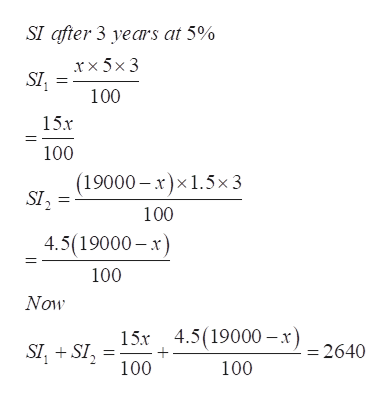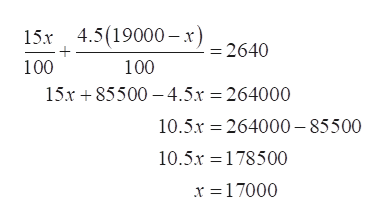Elsa  borrowed a total of \$19,000 from two different banks to start a business. one bank charges the equivalent of 5% simple interest, and the other charged 1.5% interest. If the total interest after three years  was \$2640, determine the amount borrowed from each bank.    Elsa borrowed how much at 5% and how much at 1.5%

Question

Elsa  borrowed a total of \$19,000 from two different banks to start a business. one bank charges the equivalent of 5% simple interest, and the other charged 1.5% interest. If the total interest after three years  was \$2640, determine the amount borrowed from each bank.    Elsa borrowed how much at 5% and how much at 1.5%

Step 1

Refer to the question, Elsa  borrowed a total of \$19,000 from two different banks to start a business.

Let x be the money she borrowed from one of the bank at 5% simple interest then (19000-x) is borrowed at 1.5% simple interest.

Step 2

Now the total interest after three years  was \$2640.

That is,help_outlineImage TranscriptioncloseSI after 3 years at 5% rx 5x 3 100 15x 100 (19000 x)x1.5x 3 SI2 100 4.5(19000-x 100 Now 15x 4.519000-x) = 2640 SI, SI 100 100 fullscreen
Step 3

Solve the equation to ge...help_outlineImage Transcriptionclose4.5(19000-x) - 2640 15x 100 100 15r85500 4.5.x 264000 10.5.r 264000-85500 10.5x 178500 x 17000 fullscreen

Want to see the full answer?

See Solution

Want to see this answer and more?

Our solutions are written by experts, many with advanced degrees, and available 24/7

See Solution
Tagged in

Other